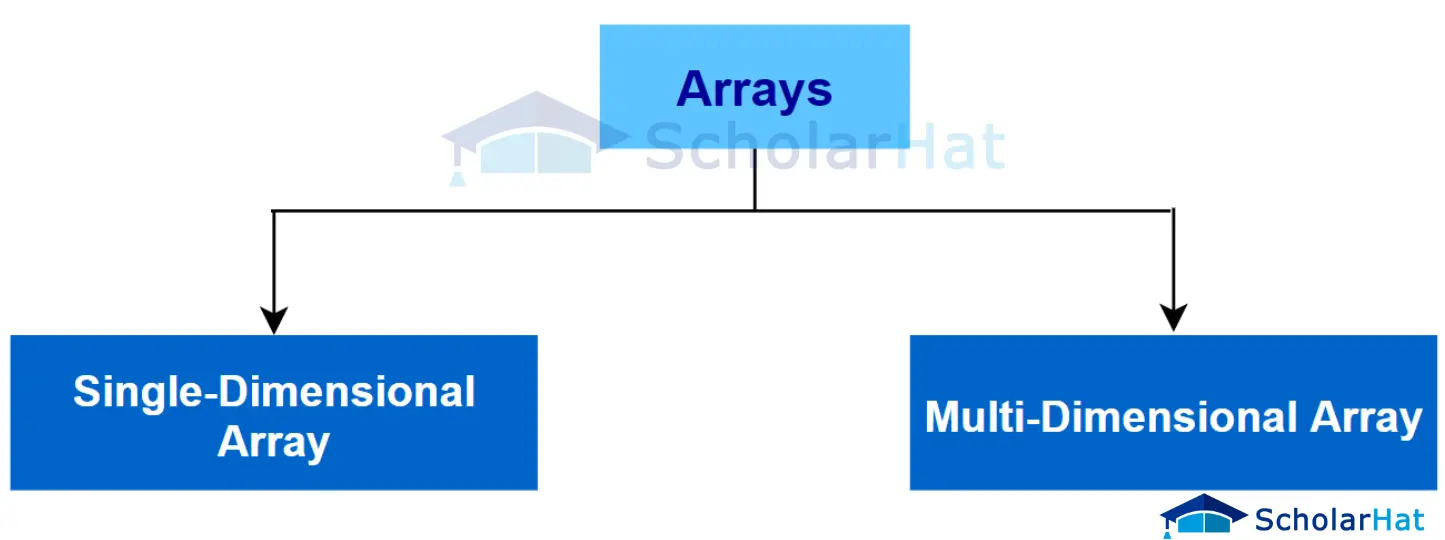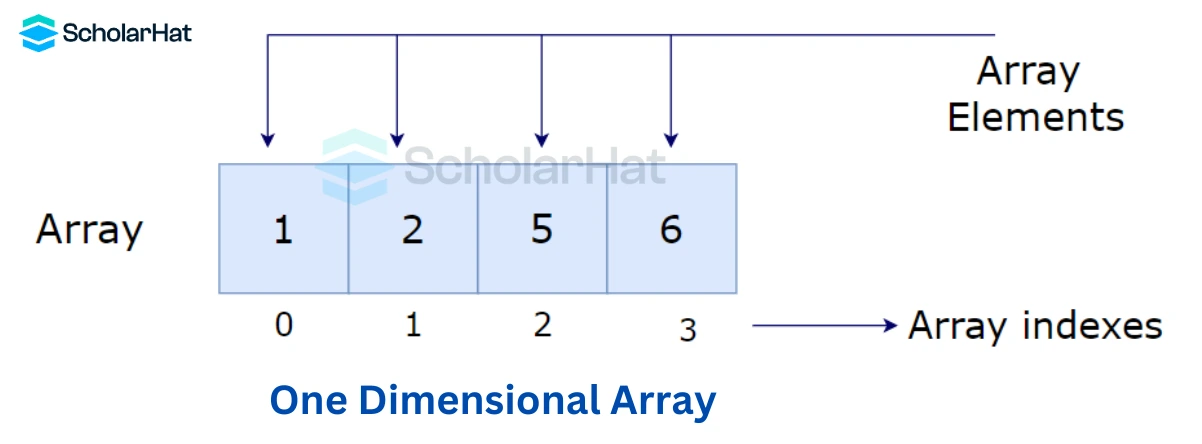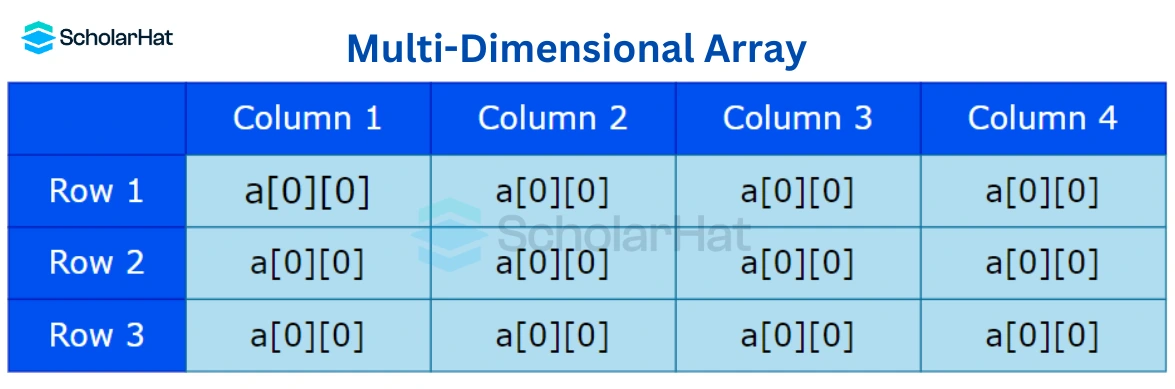# Arrays in C++: Types of Arrays

11 Sep 2023
Beginner
720 Views

## Arrays in C++: An Overview

Are you curious about one of the most versatile and powerful data structures in C++? If so, then pay attention, because Arrays are an important tool when it comes to programming, Learn more in our C++ certification courses. In this blog post, we’ll explore what makes Arrays so great: their structure, how they store information, and how they can be used in various algorithms. Read on to find out why C++ developers should know all about Arrays.

## What is an Array in C++ programming?

An Array is a group of variables with similar data types that refer to a single element. This element is stored in a contiguous memory location. The size of an Array in C++ programming should be mentioned during the process of declaration of the Array. An Array can store various primitive data types such as float, double, char, int, etc.

## Types of Array in C++

There are three types of Array in C++ programming language, are:
1. One dimensional Array
2. Two dimensional Array
3. Multi-dimensional Array

##### One-Dimensional Array in C++

One-dimensional Array in C++ makes it easier to filter, search, and show elements by storing and manipulating data effectively. They can replace dynamically allocated objects with shorter, simpler code, which enhances the robustness and memory efficiency of programs.#### One Dimensional Array in C++ Example

``````#include <iostream>
using class std;
int main()
{
int arr={10, 0, 20, 0, 30}; //creating and initializing array
//traversing array
for (int i = 0; i < 5; i++)
{
cout<<arr[i]<<"\n";
}
}
``````

This C++ code declares and initializes the five-element integer Array arr. Following that, a for loop iterates through the Array, printing each entry on a separate line. The Array's values—10, 0, 20, 0, and 30—will be shown in the output on different lines.

#### Output

``````10
0
20
0
30
``````

### Two-Dimensional Array in C++

Data in the rows and columns is represented as a two-dimensional Array in C++. A two-dimensional Array is also called a matrix. It can be of any type like integer, character, float, etc. depending on the initialization. Rows and columns are indexed starting at 0 and ending at row 1 and column 1, respectively.

#### Two Dimensional Array in C++ Example

``````#include <iostream>
int main() {
// Declare a 2D array with 3 rows and 4 columns
int myArray;
// Initialize the elements of the 2D array
for (int i = 0; i < 3; i++) {
for (int j = 0; j < 4; j++) {
myArray[i][j] = i * 4 + j + 1;
}
}
// Access and print the elements of the 2D array
std::cout << "2D Array Elements:" << std::endl;
for (int i = 0; i < 3; i++) {
for (int j = 0; j < 4; j++) {
std::cout << myArray[i][j] << " ";
}
std::cout << std::endl;
}
return 0;
}``````

This C++ code creates the 2D Array myArray, which has three rows and four columns. It initializes each element with a consecutive integer and then uses nested loops to print the contents in a grid format. The 2D Array's contents are shown in the output.

#### Output

``````2D Array Elements:
1 2 3 4
5 6 7 8
9 10 11 12 ``````

### Multi Dimensional Array in C++

With the help of Multi dimensional Array in C++, you may organize data hierarchically, increasing storage and processing flexibility. They make it possible to perform effective calculations and database searches, enabling sophisticated and dynamic solutions. These Arrays are a strong programming tool with many uses in C++.#### Multi Dimensional Array in C++ Example

``````#include <iostream>
using class std;
int main()
{
int test; //declaration of 2D array
test=5; //initialization
test=10;
test=15;
test=20;
test=30;
test=10;
//traversal
for(int i = 0; i < 3; ++i)
{
for(int j = 0; j < 3; ++j)
{
cout<< test[i][j]<<" ";
}
cout<<"\n"; //new line at each row
}
return 0;
}
``````

A 3x3 2D Array test is declared, initialized, and then traversed using this C++ code. It uses nested loops to print the full Array in a grid format while assigning specific values to some of its elements. The values of the Array items will be shown in rows and columns in the output.

#### Output

``````5 10 0
0 15 20
30 0 10
``````

## Array Initialization in C++ / Array Declaration in C++

Although they apply to separate elements of dealing with Arrays, Array initialization, & Array declaration are closely connected concepts in the C++ programming language.

### Array Declaration in C++

In C++, declaring an Array is the process of informing the compiler that it exists. It provides information to the compiler about the size and type of elements the Array will contain. The Array declaration only informs the compiler of what to expect, without allocating memory or giving values to the Array items.

#### Syntax

``data_type array_name[array_size];``
• data_type: The Array's data_type specifies the type of data it will hold (for example, int, double, char, etc.).
• array_name: The name you give your Array is array_name.
• array_size: The Array's size is its total number of elements.

#### Example

``int myArray; // Declaration of an integer array named myArray with a size of 11``

This code declares an integer Array named "myArray" with a size of 11 elements.

### Array Initialization in C++

When an Array is declared in C++, its elements are given initial values. This procedure is known as Array initialization. The Array is initialized with a set of values. You can use an initializer list to initialize an Array by enclosing values for each Array element in curly braces.

#### Syntax

``data_type array_name[array_size] = {value1, value2, ..., valueN};``
• data_type: The type of data that the Array will store is indicated by data_type.
• array_name: The name of the Array is array_name.
• array_size: The Array's size is its total number of elements.
• value1, value2, ..., valueN: An initializer list called "value1, value2,..., valueN" contains the values you intend to give the Array components.

#### Example

``int myArray = {1, 2, 3, 4, 5}; // Initialization of an integer array with values 1, 2, 3, 4, 5``

The five elements of the integer Array "myArray" that this code initializes have the values 1, 2, 3, 4, and 5.

## Accessing Array Elements in C++

Each element of an Array in C++ has a corresponding number. The value is referred to as an Array index. By using those indexes, we can access an Array's items. Square brackets [] and the index of the element you want to access are used in C++ to access the elements of an Array.

#### Syntax

``array_name[index]``
• array_name: This is the name of the Array whose elements you want to access.
• index: Access an Array's elements in C++ by using the syntax array_name[index], where the first element's index is 0, the second's index is 1, and so on.

#### Example

``````int myArray = {1, 2, 3, 4, 5}; // Example array

// Accessing elements in the array
int firstElement = myArray; // Access the first element (index 0)
int secondElement = myArray; // Access the second element (index 1)
int thirdElement = myArray; // Access the third element (index 2)
// ...
int fifthElement = myArray; // Access the fifth element (index 4)``````

This code creates the integer Array "myArray" with the numbers 1 through 5 and demonstrates how to retrieve elements using indices. MyArray represents the first element , the second by myArray, and so on.

## Why Do We Need Arrays?

• For simpler management, Arrays group related data items.
• Contiguous storage allows for efficient memory consumption.
• Appropriate for processing and iterating over data.
• Makes it possible to send data collections to functions.
• Serves as the foundation for intricate data structures.
• Simplifies programming by clearing out the mess of variables.
• Improves the readability and organization of the code.
• Important for effective data processing and algorithm creation.

## Advantages of Array in C++

• Arrays in C++ programming can give the programmer random access to the elements while using the index of that particular Array. These particular features can count as the advantages of Array in C++
• In the Array of C++ programming, it takes fewer lines of code as it creates a single array with the help of multiple elements.
• The Arrays in C++ programs are easy to access for all the elements. These particular features can count as the advantages of using Array in C++
• The method of traversal through the Array becomes easy because it uses a single loop.
• Sorting of codes in C++ programming becomes easy for using an Array as it can be accomplished by writing fewer lines of code.

## Disadvantages of Array in C++

• Unlike a linked list, an Array in a C++ program is not a dynamic Array so it allows a fixed number of declared elements to be entered at the time of declaration. These particular features can count as the disadvantages of Array in C++
• Insertion and deletion of elements in Arrays of C++ programming can be costly because the elements need to be managed accordingly as the new memory allocation.

## FAQs

### 1. What is an Array in C++ Programming Language?

A grouping of similar data type items in contiguous memory locations is referred to as an Array in C++.

### 2. What is the syntax of an Array?

A C++ Array declaration has the following syntax: data_type array_name[array_size]; where data_type denotes the type of the elements, array_name denotes what they are, and array_size indicates the number of them.

### 3. What are the 3 types of Arrays?

One-dimensional, two-dimensional, and multidimensional Arrays are the three types of Arrays available in C++.

### 4. What is the structure of an Array?

An Array's creation entails storing elements in memory sequentially and making each element accessible using an index starting at 0.

### 5. What is Array declaration?

The process of declaring an Array's data type, name, and size using the preceding syntax results in a named Array variable that is prepared for usage in a program.

##### Summary
The Array in C++ is a useful data structure for representing a sequence of elements. By using an Array, you can easily store and access data in a structurally consistent way. It is very important to understand how an Array works in C++ so that you can use it to its full potential. This will help make your code more efficient and improve your skills as a programmer. If you are not familiar with Arrays in C++, there are many resources available online that can help you get started with C++ training.
Similar Articles